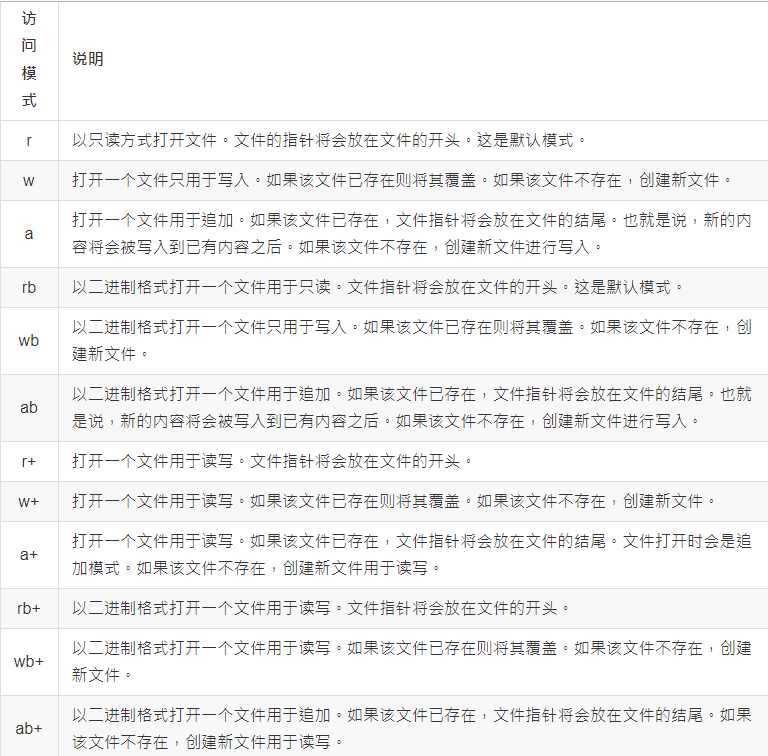Python集训营45天—Day08 (文件操作) – Python量化投资

Python集训营45天—Day08 (文件操作)

1. 文件操作介绍2. 文件的读写

import time
def main():
f = open('F://a.txt', 'r', encoding='utf-8')
f.close()
# 一次性读取整个文件的内容
with open("F://a.txt","r",encoding='utf-8') as f:

# 通过for-in循环逐行读取
with open("F://a.txt",mode="r") as f:
for line in f:
print(line,end="")

# 读取文件按行读取到列表中
with open("F://a.txt","r",encoding='utf-8') as f:
print(lines)
if __name__ == "__main__":
main()

2.1 文本文件

from math import sqrt
#是否是素数
def is_prime(n):
"""判断素数的函数"""
assert n > 0
for x in range(2,int(sqrt(n)) + 1):
if n % x == 0:
return False

return False if n == 1 else True
def main():
filenames = ('F://a.txt',"F://b.txt","F://c.txt")
fs_list = []
try:
for f in filenames:
fs_list.append(open(f,"w",encoding='utf-8'))
for number in range(1,10001):
if is_prime(number):
if number < 100:
print("1:",number)
fs_list.write(str(number) + '\n')
elif number < 1000:
print("2:",number)
fs_list.write(str(number) + '\n')
else:
print("3:",number)
fs_list.write(str(number) + '\n')
except Exception as e:
print(e)
finally:
for fs in fs_list:
fs.close()
print("操作完成")
if __name__ == '__main__':
main()

2.2 二进制文件

"""

version:0.1
author:coke
"""
def main():
try:
with open("F://aa.jpg","rb") as f1:
print(type(data))
with open("F://bb.jpg","wb") as f2:
f2.write(data)
except Exception as e:
print(e)
if __name__ == "__main__":
main()

2.3 JSON文件

"""

version:0.1
author:coke
"""
import json
def main():
mydict = {
'name': 'coke',
'age':'19',
'qq':623105972,
'friends':['David','Jack'],
'cars':[
{"brand":"BYD","max_speed":"180"},
{"brand":"BM","max_speed":"190"}
]
}
try:
with open("F://data.json","w",encoding='utf-8') as fs:
json.dump(mydict,fs)
except IOError as e:
print(e)
print("保存数据完成")
if __name__ == '__main__':
main()

json模块主要有四个比较重要的函数，分别是：

• dump – 将Python对象按照JSON格式序列化到文件中
• dumps – 将Python对象处理成JSON格式的字符串
• load – 将文件中的JSON数据反序列化成对象
• loads – 将字符串的内容反序列化成Python对象
import requests
import json
def main():
resp = requests.get("http://api.tianapi.com/guonei/?key=APIKey&num=10")
print(data_model)
print(data_model['code'])
print(data_model['msg'])
if __name__ == '__main__':
main()

3. 文件的定位，重命名和删除

seek(offset, from)有2个参数

• offset:偏移量
• from:方向
• 0:表示文件开头
• 1:表示当前位置
• 2:表示文件末尾

os模块中的rename()可以完成对文件的重命名操作，remove()可以完成对文件的删除操作

# -*- coding:utf-8 -*-
"""

version:0.1
author:coke
"""
import os
f = open("F://a.txt","r")
print(str)
#查找当前位置
position = f.tell()
print("当前位置:",position)
print(str)
#查找当前位置
position = f.tell()
print("当前位置:",position)
f.seek(1,0)
position = f.tell()
print("seek完当前位置:",position)
f.close()
#关闭文件流才可以 进行重命名和删除
os.rename("F://a.txt","F://rename.txt")
os.remove("F://b.txt")

4. 文件夹的相关操作

import os
from time import sleep
#创建文件夹
if not os.path.exists("F://tempDir"):
os.mkdir("F://tempDir")
#获取当前目录
currentDir = os.getcwd()
print(currentDir)
print("--------------")
#改变默认目录
os.chdir("F://")
#获取默认目录
defaultDir = os.listdir("./")
print(defaultDir)
#过5秒删除文件
sleep(5)
os.rmdir("F://tempDir")

https://www.cnblogs.com/dwlovelife/p/11636701.html

「点点赞赏，手留余香」

还没有人赞赏，快来当第一个赞赏的人吧！
Python
Python
0 条回复 A 作者 M 管理员
所有的伟大，都源于一个勇敢的开始！